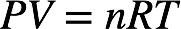# Problem: A student adds 4.00 g of dry ice (solid CO2) to an empty balloon. What will be the volume of the balloon at STP after all the dry ice sublimes (converts to gaseous CO2)?

###### FREE Expert Solution

The volume of the balloon is simply the volume occupied by CO2. We can use the ideal gas law to calculate the volume CO2 occupies. The ideal gas law is as follows:At conditions of standard temperature and pressure (STP), temperature = 273.15 K and pressure = 1 atm.

We first need to determine the number of moles of CO2. The molar mass of CO2 is 12.01 g/mol C + 2(16.00 g/mol O) = 44.01 g/mol.

100% (219 ratings)###### Problem Details

A student adds 4.00 g of dry ice (solid CO2) to an empty balloon. What will be the volume of the balloon at STP after all the dry ice sublimes (converts to gaseous CO2)?